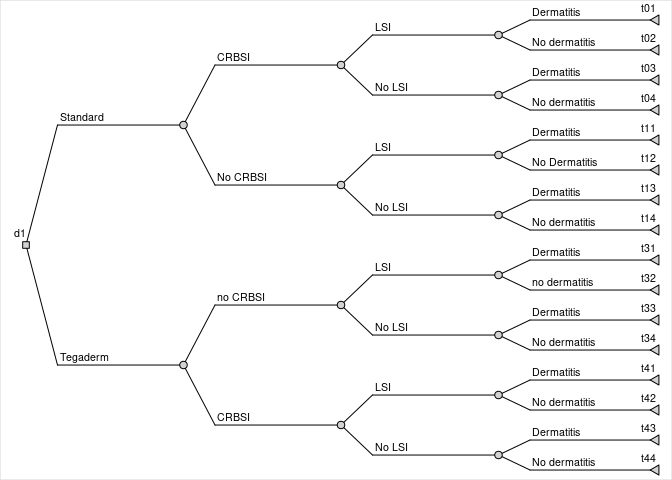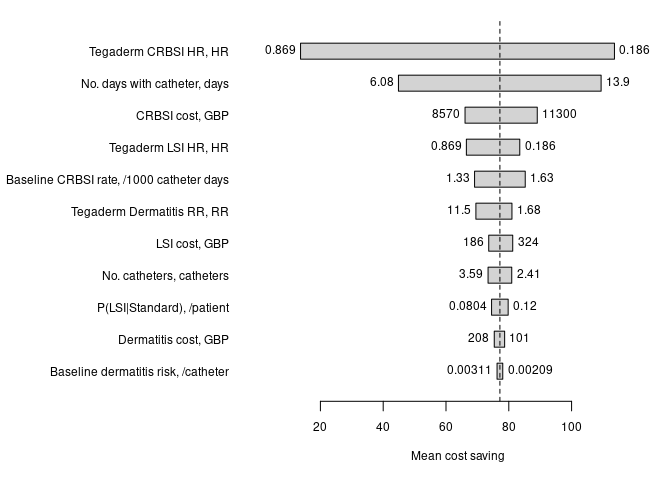# Introduction

This vignette is an example of modelling a decision tree using the rdecision package, with probabilistic sensitivity analysis (PSA). It is based on the model reported by Jenks et al1 in which a transparent dressing used to secure vascular catheters (Tegaderm CHG) was compared with a standard dressing.

# Model variables

## Source variables

Thirteen source variables were used in the model. The choice of variables, their distributions and their parameters are taken from Table 3 of Jenks et al1, with the following additional information:

• For variables with lognormal uncertainty, the company synthesized a log normal distribution as $$\exp(\mu + \sigma r())$$ where $$r()$$ is a random draw from a standard normal distribution. This is equivalent to the LN1 parametrization of the LogNormModVar provided in rdecision.
• The values for $$\mu$$ and $$\sigma$$ for the hazard ratio for CRBSI and LSI were -0.911 and -0.393 respectively, and 1.482 and 0.490 for the relative risk of dermatitis. The point estimates in the company’s model were taken at $$\exp(-0.911)$$ (0.402) and $$\exp(1.482)$$ (4.40), the expected value of the uncertainty distributions on the log scale, rather than at the expected values on the linear scale (0.434 and 4.963). In this vignette the latter are used for consistency between base case and PSA.
• The probabilities of CRBSI and LSI for standard dressings ($$p$$) were modified by the hazard ratio for Tegaderm using the form $$(1-(1-p)^r)$$ where $$r$$ is the hazard ratio. Relative risks were applied as multipliers.
• The point estimate cost of CRBSI was £9900, not £9990, although the parameters (198,50) are quoted correctly.

The model variables were constructed as follows:

# clinical variables
r.CRBSI <- NormModVar$new( 'Baseline CRBSI rate', '/1000 catheter days', mu=1.48, sigma=0.074 ) hr.CRBSI <- LogNormModVar$new(
"Tegaderm CRBSI HR", "HR", p1 = -0.911, p2 = 0.393
)
hr.LSI <- LogNormModVar$new( "Tegaderm LSI HR", "HR", p1 = -0.911, p2 = 0.393 ) r.Dermatitis <- NormModVar$new(
'Baseline dermatitis risk', '/catheter', mu=0.0026, sigma=0.00026
)
rr.Dermatitis <- LogNormModVar$new( "Tegaderm Dermatitis RR", "RR", p1=1.482, p2=0.490 ) # cost variables c.CRBSI <- GammaModVar$new(
'CRBSI cost', 'GBP', shape=198.0, scale=50
)
c.Dermatitis <- GammaModVar$new( 'Dermatitis cost', 'GBP', shape=30, scale=5 ) c.LSI <- GammaModVar$new(
'LSI cost', 'GBP', shape=50, scale=5
)
n.catheters <- NormModVar$new( 'No. catheters', 'catheters', mu=3, sigma=0.3 ) c.Tegaderm <- ExprModVar$new(
"Tegaderm CHG cost", "GBP", rlang::quo(6.21*n.catheters)
)
c.Standard <- ExprModVar$new( "Standard dressing cost", "GBP", rlang::quo(1.34*n.catheters) ) n.cathdays <- NormModVar$new(
'No. days with catheter', 'days', mu=10, sigma=2
)  

## Model variable expressions

Variables in the model may be included in the decision tree via mathematical expressions, which involve model variables and are themselves model variables. Forms of expression involving R functions and multiple model variables are supported, provided they conform to R syntax. The following code creates the model variable expressions to be used as values in the decision tree edges. For probabilities, the convention $$q = 1-p$$ is used to ensure that the sum of probabilities leaving each chance node is one.

# probabilities
p.Dermatitis.S <- ExprModVar$new( 'P(dermatitis|standard dressing)', 'P', rlang::quo(r.Dermatitis*n.catheters) ) q.Dermatitis.S <- ExprModVar$new(
'Q(dermatitis|standard dressing)', '1-P',
rlang::quo(1-p.Dermatitis.S)
)
p.Dermatitis.T <- ExprModVar$new( 'P(dermatitis|Tegaderm)', 'P', rlang::quo(r.Dermatitis*rr.Dermatitis*n.catheters) ) q.Dermatitis.T <- ExprModVar$new(
rlang::quo(1-p.Dermatitis.T)
)

p.LSI.S <- NormModVar$new( 'P(LSI|Standard)', '/patient', mu=0.1, sigma=0.01 ) q.LSI.S <- ExprModVar$new(
'Q(LSI|Standard)', '1-P', rlang::quo(1-p.LSI.S)
)
p.LSI.T <- ExprModVar$new( 'P(LSI|Tegaderm)', 'P', rlang::quo(1-(1-p.LSI.S)^hr.LSI) ) q.LSI.T <- ExprModVar$new(
)

p.CRBSI.S <- ExprModVar$new( 'P(CRBSI|standard dressing)', 'P', rlang::quo(r.CRBSI*n.cathdays/1000) ) q.CRBSI.S <- ExprModVar$new(
'Q(CRBSI|standard dressing)', '1-P',  rlang::quo(1-p.CRBSI.S)
)
p.CRBSI.T <- ExprModVar$new( 'P(CRBSI|Tegaderm)', 'P', rlang::quo(1-(1-r.CRBSI*n.cathdays/1000)^hr.CRBSI) ) q.CRBSI.T <- ExprModVar$new(
)

# The decision tree

## Constructing the tree

The following code constructs the decision tree based on Figure 2 of Jenks et al1. In the formulation used by rdecision, the decision tree is constructed from sets of decision, chance and leaf nodes and from edges (actions and reactions). Leaf nodes are synonymous with pathways in Briggs’ terminology2. The time horizon is not stated explicitly in the model, and is assumed to be 7 days. It was implied that the time horizon was ICU stay plus some follow-up, and the costs reflect those incurred in that period, so the assumption of 7 days does not affect the rdecision implementation of the model.

The tree is somewhat more complex than Figure 2 of Jenks et al because it allows for patients to have more than one adverse event (AE) during their stay (whereas their Figure 2 implies that only one event per patient is possible). The rates of AE were estimated independently, and allow for multiple events, see figure.

# create decision tree
th <- as.difftime(7, units="days")
# standard dressing
t01 <- LeafNode$new("t01", interval=th) t02 <- LeafNode$new("t02", interval=th)
c01 <- ChanceNode$new() e01 <- Reaction$new(c01,t01,p=p.Dermatitis.S,cost=c.Dermatitis,
label="Dermatitis")
e02 <- Reaction$new(c01,t02,p=q.Dermatitis.S,cost=0, label="No dermatitis") # t03 <- LeafNode$new("t03", interval=th)
t04 <- LeafNode$new("t04", interval=th) c02 <- ChanceNode$new()
e03 <- Reaction$new(c02,t03,p=p.Dermatitis.S,cost=c.Dermatitis, label="Dermatitis") e04 <- Reaction$new(c02,t04,p=q.Dermatitis.S,cost=0,
label="No dermatitis")
#
c03 <- ChanceNode$new() e05 <- Reaction$new(c03,c01,p=p.LSI.S,cost=c.LSI,label="LSI")
e06 <- Reaction$new(c03,c02,p=q.LSI.S,cost=0,label="No LSI") # t11 <- LeafNode$new("t11", interval=th)
t12 <- LeafNode$new("t12", interval=th) c11 <- ChanceNode$new()
e11 <- Reaction$new(c11,t11,p=p.Dermatitis.S,cost=c.Dermatitis, label="Dermatitis") e12 <- Reaction$new(c11,t12,p=q.Dermatitis.S,cost=0,label="No Dermatitis")
#
t13 <- LeafNode$new("t13", interval=th) t14 <- LeafNode$new("t14", interval=th)
c12 <- ChanceNode$new() e13 <- Reaction$new(c12,t13,p=p.Dermatitis.S,cost=c.Dermatitis,
label="Dermatitis")
e14 <- Reaction$new(c12,t14,p=q.Dermatitis.S,cost=0,label="No dermatitis") # c13 <- ChanceNode$new()
e15 <- Reaction$new(c13,c11,p=p.LSI.S,cost=c.LSI,label="LSI") e16 <- Reaction$new(c13,c12,p=q.LSI.S,cost=0,label="No LSI")
#
c23 <- ChanceNode$new() e21 <- Reaction$new(c23,c03,p=p.CRBSI.S,cost=c.CRBSI,label="CRBSI")
e22 <- Reaction$new(c23,c13,p=q.CRBSI.S,cost=0,label="No CRBSI") # # Tegaderm branch t31 <- LeafNode$new("t31", interval=th)
t32 <- LeafNode$new("t32", interval=th) c31 <- ChanceNode$new()
e31 <- Reaction$new(c31,t31,p=p.Dermatitis.T,cost=c.Dermatitis, label="Dermatitis") e32 <- Reaction$new(c31,t32,p=q.Dermatitis.T,cost=0,label="no dermatitis")
#
t33 <- LeafNode$new("t33", interval=th) t34 <- LeafNode$new("t34", interval=th)
c32 <- ChanceNode$new() e33 <- Reaction$new(c32,t33,p=p.Dermatitis.T,cost=c.Dermatitis,
label="Dermatitis")
e34 <- Reaction$new(c32,t34,p=q.Dermatitis.T,cost=0,label="No dermatitis") # c33 <- ChanceNode$new()
e35 <- Reaction$new(c33,c31,p=p.LSI.T,cost=c.LSI,label="LSI") e36 <- Reaction$new(c33,c32,p=q.LSI.T,cost=0,label="No LSI")
#
t41 <- LeafNode$new("t41", interval=th) t42 <- LeafNode$new("t42", interval=th)
c41 <- ChanceNode$new() e41 <- Reaction$new(c41,t41,p=p.Dermatitis.T,cost=c.Dermatitis,
label="Dermatitis")
e42 <- Reaction$new(c41,t42,p=q.Dermatitis.T,cost=0,label="No dermatitis") # t43 <- LeafNode$new("t43", interval=th)
t44 <- LeafNode$new("t44", interval=th) c42 <- ChanceNode$new()
e43 <- Reaction$new(c42,t43,p=p.Dermatitis.T,cost=c.Dermatitis, label="Dermatitis") e44 <- Reaction$new(c42,t44,p=q.Dermatitis.T,cost=0,label="No dermatitis")
#
c43 <- ChanceNode$new() e45 <- Reaction$new(c43,c41,p=p.LSI.T,cost=c.LSI,label="LSI")
e46 <- Reaction$new(c43,c42,p=q.LSI.T,cost=0,label="No LSI") # c53 <- ChanceNode$new()
e51 <- Reaction$new(c53,c43,p=p.CRBSI.T,cost=c.CRBSI,label="CRBSI") e52 <- Reaction$new(c53,c33,p=q.CRBSI.T,cost=0,label="no CRBSI")
#
# decision node
d1 <- DecisionNode$new("d1") e9 <- Action$new(d1,c23,label="Standard",cost=c.Standard)
e10 <- Action$new(d1,c53,label="Tegaderm",cost=c.Tegaderm) # # create decision tree V <- list(d1,c01,c02,c03,c11,c12,c13,c23,c31,c32,c33,c41,c42,c43,c53, t01,t02,t03,t04,t11,t12,t13,t14,t31,t32,t33,t34,t41,t42,t43,t44) E <- list(e01,e02,e03,e04,e05,e06,e11,e12,e13,e14,e15,e16,e21,e22, e31,e32,e33,e34,e35,e36,e41,e42,e43,e44,e45,e46,e51,e52,e9,e10) DT <- DecisionTree$new(V,E)

## Tree diagramDecision tree for the Tegaderm model

In the company’s model, the uncertainties in the probabilities associated with the polytomous chance nodes were modelled as independent variables. This is not recommended because there is a chance that a particular run of the PSA will yield probabilities that are outside the range [0,1].

## Summary of model variables in the tree

The model variables which will be associated with actions, reactions and leaf nodes can be tabulated using the method modvar_table. This returns a data frame describing each variable, its description, units and uncertainty distribution. Variables inheriting from type ModVar will be included in the tabulation unless explicitly excluded, regular numeric values will not be listed. In the Tegaderm model, the input model variables are in the following table, with expression model variables excluded.

Description Distribution
Dermatitis cost Ga(30,5)
Baseline dermatitis risk N(0.0026,0.00026)
No. catheters N(3,0.3)
LSI cost Ga(50,5)
P(LSI|Standard) N(0.1,0.01)
CRBSI cost Ga(198,50)
Baseline CRBSI rate N(1.48,0.074)
No. days with catheter N(10,2)
Tegaderm Dermatitis RR LN(1.482,0.49)
Tegaderm LSI HR LN(-0.911,0.393)
Tegaderm CRBSI HR LN(-0.911,0.393)

## Point estimates and distributions of model variables

The point estimates, units and distributional properties are obtained from the same call, in the remaining columns.

Variable Mean Q2.5 Q97.5
Dermatitis cost, GBP 150 101 208
Baseline dermatitis risk, /catheter 0.0026 0.00209 0.00311
No. catheters, catheters 3 2.41 3.59
LSI cost, GBP 250 186 324
P(LSI|Standard), /patient 0.1 0.0804 0.12
CRBSI cost, GBP 9900 8569 11326
Baseline CRBSI rate, /1000 catheter days 1.48 1.33 1.63
No. days with catheter, days 10 6.08 13.9
Tegaderm Dermatitis RR, RR 4.96 1.68 11.5
Tegaderm LSI HR, HR 0.434 0.186 0.869
Tegaderm CRBSI HR, HR 0.434 0.186 0.869

# Running the model

## Base case

The following code runs a single model scenario, using the evaluate method of a decision node to evaluate each pathway from the decision node, shown in the table. This model did not consider utility, and the columns associated with utility are removed.

Run d1 Cost
1 Standard 176.7

## Univariate sensitivity analysis

The sensitivity of the decision tree results to each source model variable, varied independently of the others, is demonstrated by a tornado diagram. The method tornado can be used to generate such a plot (and also provides a tabulated version of the values used in the plot). Source variables are varied over their 95% confidence limits, see figure.## Probabilistic sensitivity analysis

Multivariate probabilistic sensitivity analysis is supported through the use of sampling model variables. The same call, with extra parameters, is used to run the PSA:

N <- 1000
PSA <- DT\$evaluate(setvars="random", by="run", N=N)

The first few runs of PSA are as follows; the by="run" option reshapes the table to give one row per simulation, rather than one row per run, per strategy.

Run Cost.Tegaderm Cost.Standard Difference
1 76.34 205.2 -128.8
2 110.8 164.4 -53.65
3 117.7 215.6 -97.92
4 107 204.1 -97.14
5 108.3 181.8 -73.54
6 84.23 229.9 -145.7
7 95.24 194.7 -99.49
8 91.57 161.9 -70.35
9 158.3 145.7 12.62
10 138.1 157.7 -19.65

From PSA (1000 runs), the mean cost of treatment with Tegaderm was 99.06, the mean cost of treatment with standard dressings was 175.7 and the mean cost saving was -76.64. The 95% confidence interval for cost saving was -141.14 to -13.15; the standard deviation of the cost saving was 32.38. Overall, 98.9% of runs found that Tegaderm was cost saving. These results replicate those reported by the manufacturer (saving of 77.76, 98.5% cases cost saving; mean cost of standard dressing 176.89, mean cost of Tegaderm 99.63).